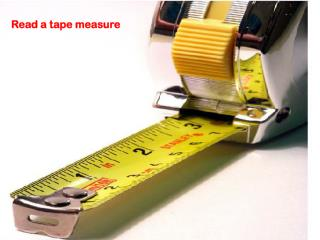# Read a tape measure - PowerPoint PPT PresentationDownload PresentationDownload Presentation- - - - - - - - - - - - - - - - - - - - - - - - - - - E N D - - - - - - - - - - - - - - - - - - - - - - - - - - -
##### Presentation Transcript

2. Measuring tools • Tape measure • Ruler • Yard stick • Folding ruler • Measuring wheel • Scale ruler

3. QUESTIONS • Who wants to maximize their proficiency? • Which tool do you think is a primary measuring tool in the Automotive field? • Today you are going to learn how to use a ruler to make proper measurements in whole and fractional units.

4. FRACTIONS OF AN INCH HALF INCH INCREMENTS http://www.rickyspears.com/rulergame/

5. FRACTIONS OF AN INCH One-quarter inch measures

6. FRACTIONS OF AN INCH One-eighth of an inch

7. FRACTIONS OF AN INCH Two-sixteenths of an inch or One-eighth of an inch

8. FRACTIONS OF AN INCH Three-sixteenths of an inch

9. FRACTIONS OF AN INCH Four-sixteenths of an inch or Two-eighths of an inch or One-fourth of an inch

10. FRACTIONS OF AN INCH Five-sixteenths of an inch

11. FRACTIONS OF AN INCH Six-sixteenths of an inch or Three –eights of an inch

12. FRACTIONS OF AN INCH Seven-sixteenths of an inch

13. FRACTIONS OF AN INCH Eight-sixteenths of an inch or Four-eighths of an inch or Two-fourths of an inch or One-half of an inch

14. FRACTIONS OF AN INCH Nine-sixteenths of an inch

15. FRACTIONS OF AN INCH Ten-sixteenths of an inch or Five-eighths of an inch

16. FRACTIONS OF AN INCH Eleventh-sixteenths of an inch

17. FRACTIONS OF AN INCH Twelve-sixteenths of an inch or Six-eighths of an inch or Three-fourths of an inch

18. FRACTIONS OF AN INCH Thirteen-sixteenths of an inch

19. FRACTIONS OF AN INCH Fourteen-sixteenths of an inch or Seven-eighths of an inch

20. FRACTIONS OF AN INCH Fifteen-sixteenths of an inch

21. FRACTIONS OF AN INCH Sixteen-sixteenths of an inch or One inch

23. FRACTION RULES RULES 1. To add fractions you must have a common denominator 2. Reduce fractions

24. COMMON DENOMINATOR How do you get a common denominator? 8 can be the common denominator when the fractions are in fourths or eighths. 16can be the common denominator for all rulers marked in 16ths. Use 32as the common denominator when the ruler is marked in 32nds.

25. COMMON DENOMINATOR Multiply top and bottom by the same value to change the denominator correctly.

26. ADDING MEASURES • Get the common denominator • Rewrite the fractions

27. ADDING MEASURES • Get the common denominator • Rewrite the fractions • Add the numerators • Carry the denominator forward

28. ADDING MEASURES • Get the common denominator • Rewrite the fractions • Add the numerators • Carry the denominator forward • Reduce the answer

29. ADDING MEASURESwith WHOLE NUMBERS • Add the whole numbers (like always) • Get the common denominator • Rewrite the fractions • Add the numerators • Carry the denominator forward • Reduce the answer

32. REMEMBERCOMMON DENOMINATORADDING & SUBTRACTING How do you get a common denominator? 8 can be the common denominator when the fractions are in fourths or eighths. 16can be the common denominator for all rulers marked in 16ths. Use 32as the common denominator when the ruler is marked in 32nds.

33. COMMON DENOMINATOR Multiply top and bottom by the same value to change the denominator correctly.

34. SUBTRACTING MEASURES • Get the common denominator • Rewrite the fractions

35. SUBTRACTING MEASURES • Get the common denominator • Rewrite the fractions • Subtract the numerators • Carry the denominator forward

36. SUBTRACTING MEASURES • Get the common denominator • Rewrite the fractions • Subtract the numerators • Carry the denominator forward • Reduce the answer

37. SUBTRACTING MEASURESwith WHOLE NUMBERS • Subtract the whole numbers (like always) • Get the common denominator • Rewrite the fractions • Subtract the numerators • Carry the denominator forward • Reduce, if necessary

38. SUBTRACTING MEASURES

39. SUBTRACTING MEASURES

40. SUBTRACTING MEASURES

41. MULTIPLYING MEASURES • One-half of 1 inch means or of 1 inch “of is another way to say times”

42. MULTIPLYING MEASURES Two times one-half is adding one-half two times. 2 X  = 1 +  =1